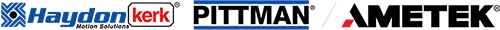# Temperature Effects on DC Motor Performance

When applying DC motors to any type of application, temperature effects need to be considered in order to properly apply the motor. Performance will change as the motor temperature changes. When reviewing DC motor curves, the user needs to ask the question “Do these curves represent performance of the motor at room temperature, or do these curves illustrate performance at the maximum rated temperature?” Depending on the temperature and the required operating point on the motor curve, the performance difference between “cold” and “hot” conditions can be significant.

Once a motor design is finalized including motor dimensions, magnetic circuit, and motor winding configuration, several characteristics that determine motor performance become “theoretically” fixed; the torque constant (KT), voltage constant (KE), and motor terminal resistance (Rmt). These three values will determine the output torque, motor speed, and the resulting output power at any point on the motor curve at a given terminal voltage, as well as the overall slope of the motor curve.Motor Constants Aren’t REALLY Constant

The torque constant and voltage constant are determined during the design phase and is a function of the overall magnetic circuit design. They are always equal when using SI units. For example, if the motor KT = 0.1 Nm/A, then motor KE = 0.1 V/(rad/s) assuming Nm and V/(rad/s) are the units used. Motor terminal resistance is also determined in the design phase by the number of coils, number of coil turns, and magnet wire diameter. These principles apply to both brush and brushless DC motors.

The phrase “motor constants”, however, is somewhat of a misnomer. Winding resistance and permanent magnet flux density will change as temperature changes. As the motor temperature increases, winding resistance will increase based on the temperature coefficient of copper. The flux density of the permanent magnets will also decrease as a function of temperature. Changes in these 2 key components of the motor will result in an increase in motor no-load speed and a decrease in motor locked rotor torque altering the overall slope of the motor curve. Once the user understands this concept, it becomes clear that motor performance determined using a rapid dynamometer test with a room temperature motor is significantly different than the motor performance when operated at its maximum temperature under load.The DC Motor Curve
DC motor performance curves can be generated under various conditions. For example, the motor curve illustrated in Figure 1 is representative of an open-loop motor tested rapidly (to prevent heating) on a dynamometer. The test would be done by quickly loading the motor from no-load to locked rotor (stall) using a fixed terminal voltage from a power supply with low output impedance. A test like this is done in order to get a “baseline” measure of motor performance while the motor is at room temperature. Motor shaft speed and current are plotted as a function of motor torque. From this test and a few resistance readings, the torque constant, voltage constant, and terminal resistance values can be determined. This information is very useful to ballpark basic motor performance and can be sufficient for an application that requires intermittent operation with a long rest period between each duty cycle.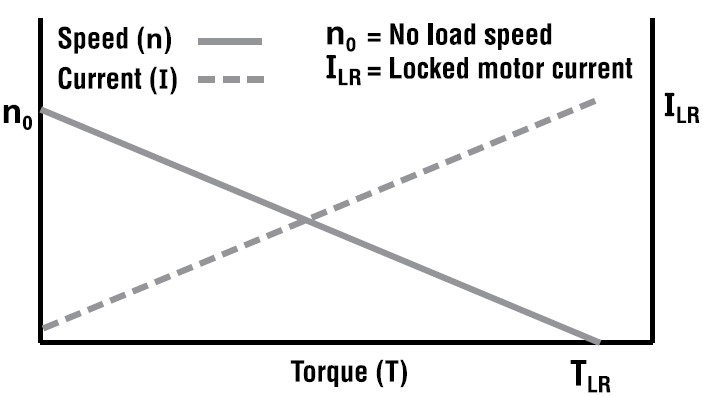Figure 1 Typical DC Motor Curve: Rapid Performance Test

In applications such as repeated point-to-point moves, applications requiring frequent starting and stopping of a high inertia load, or applications that require the motor to be moving for long periods of time (such as a fan application) the motor data illustrating performance at room temperature is not adequate and can result in misapplication of the motor or exceeding the motor’s maximum temperature rating.

As the motor temperature increases, the resistance will increase and the torque constant and voltage constant will decrease. This results in an increase in no-load speed and a decrease in locked-rotor torque. Figure 2 illustrates an example of both “cold” and “hot” running conditions of the same DC motor. The “hot” motor curve demonstrates how much the performance can change when operating the motor at an elevated temperature.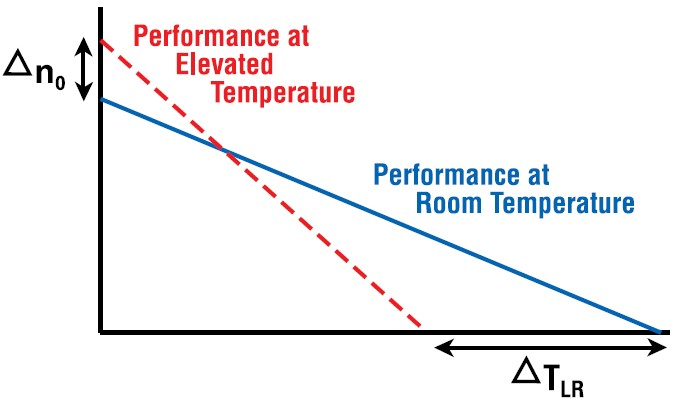Figure 2 Performance: Room Temperature and Elevated TemperatureTemperature Effects of Motor Winding Resistance
Motor winding resistance (Rmt) is the main cause of heat generation within the motor. In order for any electric motor to generate torque, current needs to be forced through the motor windings. Copper is an excellent conductor, however, it’s not perfect; material physics and impurities will cause the atoms to vibrate at a faster rate as more current flows. The result is a steady temperature increase in the motor windings. All metal conductors have a positive temperature coefficient of resistance. This means as temperature increases, the resistance of the material also increases as a function of the type of conductor used. Electric motors typically use copper conductor material, except in special cases. Many induction motor squirrel cages use cast aluminum for ease of manufacturing, but the vast majority of motors use copper magnet wire. Table 2 lists examples of common metals used in electrical and electronic devices and their respective temperature coefficients (α).

 Table 1. Symbols and UnitsTable 2. Temperature Coefficients for Various Materials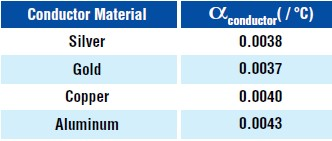Equations 1 and 2 illustrate the relationship between winding temperature, winding resistance, and watts dissipated.Equation 1. Change in Winding ResistanceEquation 2. Watts Lost Due to Winding ResistanceTemperature Effects On Magnetic Flux Density
The motor torque constant (KT) and voltage constant (KE) are directly related to the magnetic flux density (Br) of the permanent magnets. Depending on the physics of the magnet material used, overall flux density will change at a given percentage with an increase in magnet temperature. As the material temperature increases, atomic vibrations cause once-aligned magnetic moments to “randomize” resulting in a decrease in magnetic flux density. Assuming the motor is operating within its intended design window, the decrease in flux density is temporary and will begin to recover as the magnet cools. If the maximum temperature rating of the magnets is exceeded, however, partial demagnetization will occur and permanently alter the performance of the motor.

The values in Table 3 represent average figures for material classes. Specific magnet grades within a class of materials will vary from the values given below. For example, some grades of Neodymium magnets can exceed the listed operating temperature of 150°C. The table illustrates relative differences between temperature characteristics of various materials. If exact values are needed, it’s recommended to consult an application engineer at the motor manufacturer for specific information.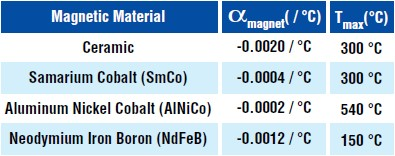Table 3. Temperature Coefficients for Various Permanent Magnet MaterialsEquation 3. Change in KT and KE ( KT = KE when using SI Units )

A question often asked by users is the relationship between KT and KE as a result of elevated temperature. In the case of using SI units, one will always equal the other and both will change equally with temperature.

In the case of using English units, KT and KE are expressed in oz-in/A (lb-in/A, lb-ft/A, etc.) and V/krpm. One does not equal the other when using these units, but both quantities will decrease in the same proportion with elevated temperature.

How does this affect overall motor performance?
At an elevated motor temperature, the slope of the DC motor curve increases as a result of an increase in no-load speed and a decrease in locked rotor torque (sometimes referred to as stall torque). Figure 3 below illustrates the total area beneath a particular motor curve that can be considered the range of consistent performance between room temperature and maximum rated motor temperature.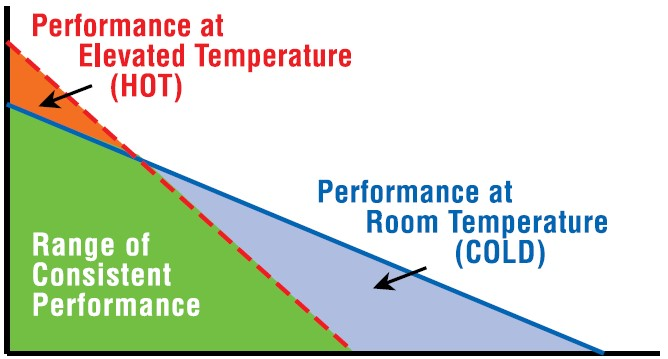Figure 3. Motor Performance: "Unusable" Area Under Motor Performance Curve

Another term referring to the slope of the DC motor curve is “regulation”. Motor regulation describes how much the shaft speed will change with a given change in shaft load when applying a fixed terminal voltage to the motor (operating as an open loop system). A flatter speed-torque curve will result in a smaller change in shaft speed with increased load. As the motor temperature increases and the curve becomes steeper, shaft speed will drop more for the same increase in load.

 Equation 4a. Theoretical Motor Regulation Using ConstantsEquation 4b. Motor Regulation Using A Performance CurveEquations 4 and 5 can be used to illustrate the dramatic effect on motor performance resulting from a decrease in magnetic flux and increase in winding resistance. Although there will be an increase in motor no-load speed as well as a decrease in locked rotor torque, the difference in “cold” versus “hot” locked rotor torque is greater compared with the difference in “cold” versus “hot” no-load speed.

The locked rotor torque is heavily dependent on both the resistance (Rmt) and torque constant (KT). Although the no load speed will also be affected by the higher resistance at an elevated temperature, the resistance factor has a much smaller effect on no-load speed because it’s multiplied by the no load current (I0), a relatively small number (compare equation 5b and 5c).

 Equation 5a. Motor Locked Rotor (Stall) CurrentEquation 5b. Motor Locked Rotor (Stall) TorqueEquation 5c. Motor No Load Speed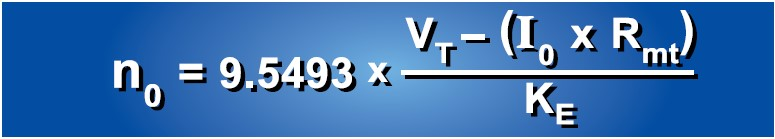The user needs to be careful when designing the system to insure the shaft speed meets the minimum specification at a given load at an elevated motor temperature. In a more complex motion system using a closed-loop controller, shaft speed can be controlled within a given range to remain “fixed” as the load requirements change. The example that follows assumes the motor is operated open-loop with a regulated DC terminal voltage.

How much can motor performance change under a given set of conditions?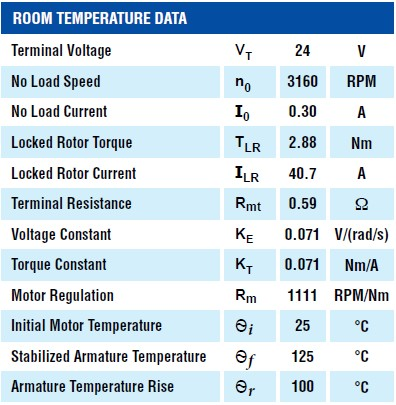Conditions: Initial motor data was created using a rapid dynamometer test at a room temperature of 25°C With a consistent load on the motor, the armature temperature stabilized to 125°C No heat sink or forced air flow was used The motor was operated open-loop (no feedback to regulate shaft output power) Power supply used – regulated, 50A max output with low output impedance Copper motor windings Strontium ferrite (ceramic) permanent magnets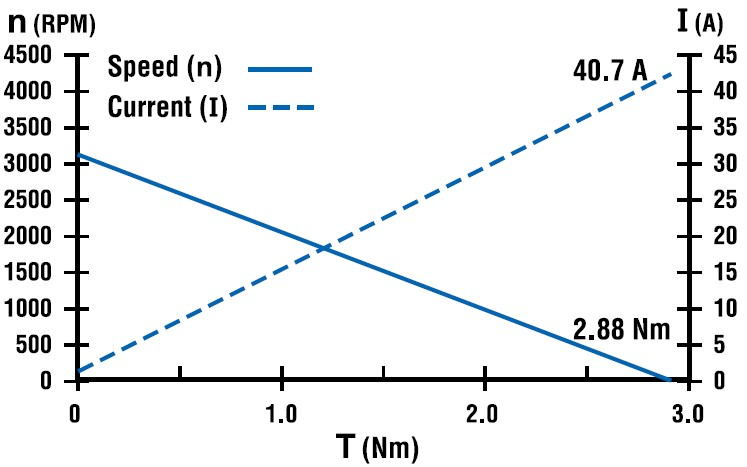Figure 4. Initial Motor Performance @ 25°C

With a stabilized armature temperature rise of 100°C, terminal resistance will be higher and magnetic flux density will be lower compared with the initial motor temperature of 25°C. This will result in a change in Rmt, KT, and KE.

Important Note – The calculations that follow assume that the permanent magnet and wound armature temperatures are identical. The motor armature, which is the part of the machine carrying electric current, will always be at a higher temperature than the permanent magnets. In practice, the actual change in KT, KE, and motor regulation will be less pronounced than what’s demonstrated in the following example.Estimated Motor Characteristics at an Elevated Temperature of 125°C

 Increased Terminal Resistance Rmt( f ) = Rmt(i) x [1 + αconductor (Ѳf - Ѳi)] Rmt( f ) = 0.59Ω x [1 + 0.0040 (125°C - 25°C)] Rmt( f ) = 0.83Ω Decreased Torque and Voltage Constant (K used for both KE and KT assuming SI units) K( f ) = K(i) x [1 + αmagnet (Ѳf - Ѳi)] K( f ) = 0.071 V/(rad/s) x [1 + (-0.0020)(125°C - 25°C)] K( f ) = 0.057 V/(rad/s) or Nm/A Decreased Locked Rotor (Stall) Current ILR = VT / Rmt( f ) ILR = 24V / 0.83Ω ILR = 28.92A Decreased Locked Rotor (Stall) Torque TLR = ILR x KT( f ) TLR = 28.92A x 0.057Nm/A TLR = 1.65Nm Increased No Load Speed n0 = 9.5493 x [(VT – I0 x Rmt( f )) / KE( f )] n0 = 9.5493 x [(24V – 0.30A x 0.83 Ω) / 0.057V/(rad/s)] n0 = 3979 RPM Increased Motor Regulation Rm = n0 / TLR Rm = 3979 RPM / 1.65 Nm Rm = 2412 RPM / Nm

 Table 4. Change in Motor Characteristics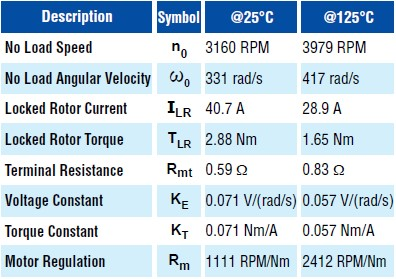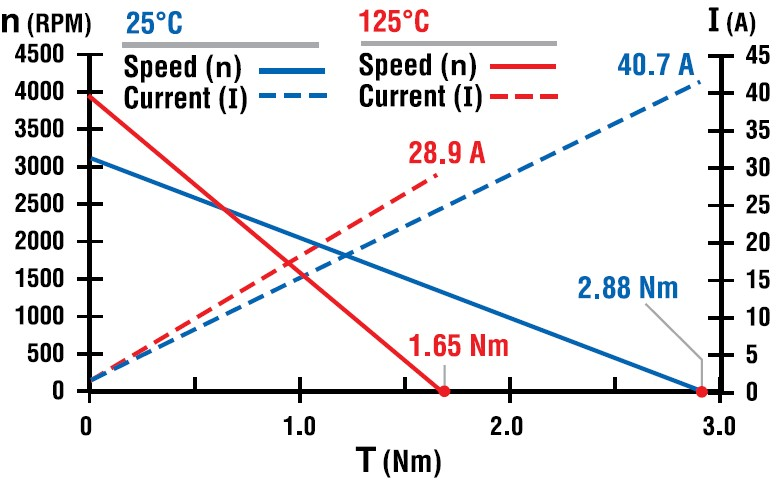Figure 5. Motor Performance Comparison @ 25°C and 125°C

It’s interesting to note how much the performance changes with a given increase in motor temperature. Although the analysis is helpful in understanding the phenomenon, it’s by no means perfect. There are a few things to keep in mind, for example...

 We assumed a stabilized 100°C rise on all components of the motor. In practice, the various motor components will stabilize at different temperatures, with the wound motor armature being the highest. The temperature coefficient for the magnetic material was an average used for that material. In reality, different grades of a particular material class will have values that deviate slightly from the average. The true thermal model of a DC motor is extremely complex. It’s very difficult to obtain exact values theoretically, as there are just too many variables to consider.
The preceding first approximation analysis however, is very helpful in applying the motor and understanding its limitations in a particular application.Motor Constants and Maximum Output Power
Rarely, if ever, is a motor operated at the maximum power point for very long. With the exception of very small, low power motors, most cannot be operated continuously at the maximum power point without exceeding the temperature rating. The equations below can be used to determine the power at any point on the motor curve
and maximum output power.

 Equation 6a. Motor Output Power (at any point)Equation 6b. Motor Maximum Output PowerEquation 6c. Motor Maximum Output Power (Theoretical)Equations 6b and 6c illustrate the effect of motor resistance on the output power capability of the machine. Although not intuitive by looking at equation 6b, a higher winding resistance at an elevated temperature is the main culprit in reducing the maximum output power capability of the motor. Even though magnetic flux density is reduced at an elevated temperature, the decrease in TLR (due to reduced KT) is compensated by an increase in ω0 (due to reduced KE). If the resistance stayed the same, even a decrease in magnetic flux density will not significantly change the maximum output power of the machine.

In reality, the higher resistance (Rmt) combined with the reduced magnetic flux density (lower KT) reduces the locked rotor torque much more than just a reduction in magnetic flux density alone, which accounts for the reduction in maximum output power.

 Maximum output power @ 25°C Pmax(i) = 0.25 x ω0 x TLR Pmax(i) = 0.25 x 331 rad/s x 2.88 Nm Pmax(i) = 238 W Maximum output power @ 125°C Pmax( f ) = 0.25 x ω0 x TLR Pmax( f ) = 0.25 x 417 rad/s x 1.65 Nm Pmax( f ) = 172 W

The maximum power at the elevated temperature is approximately 70% of the maximum power at room temperature. This is a significant change, and it’s a  direct consequence of the increased motor resistance. Equation 6c will give results that are within 1 or 2% of the numbers above. This equation is very helpful in rapidly estimating the maximum power capability of any DC motor.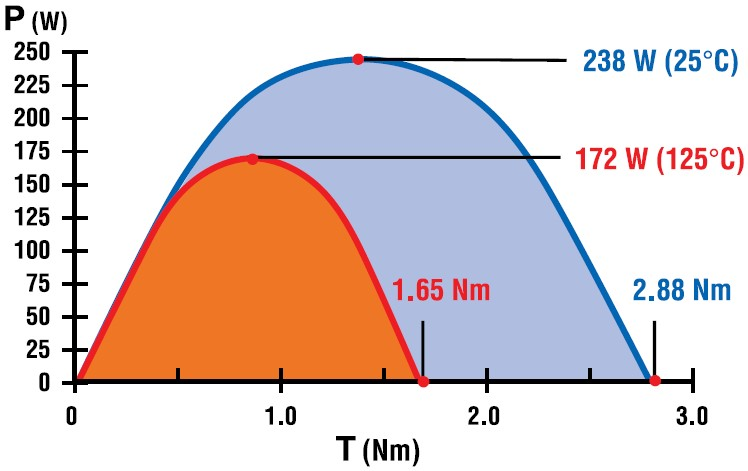Figure 6. Maximum Power Capacity @ 25°C and 125°CPublished Data – Motor curves come in many varieties!
Most DC motor performance charts will show at least 2 curves; speed versus torque and current versus torque. Manufacturers may decide to display the information in slightly different ways and also supply other information such as output power and efficiency curves.

The example motor curve as shown in Figure 1 removes all other variables and assumes the motor is tested with a fixed terminal voltage using a low impedance power supply and progressively loaded with a dynamometer. The test is performed as quickly as possible to minimize motor temperature rise. If the motor being tested is a small sub-fractional horsepower motor, the dynamometer may load the motor until shaft speed hits zero or close to zero RPM. In the case of larger motors, the dynamometer may be set to progressively load the motor to approximately the maximum power point. The remainder of the curve may then be extrapolated. The resulting data in either case are (theoretically) linear speed versus torque and current versus torque curves.

Some manufacturers, in particular those that supply complete servo systems, may present performance curves as a “blend” of information that includes system-level limitations. The limitations considered can include many things such as continuous current capability, peak current capability, drive/amplifier power limitations, maximum dc bus voltage, maximum motor temperature ratings, motor saturation, and mechanical speed limitations. Many manufacturers may illustrate motor torque on the “Y” axis and speed on the “X” axis. The example curves in Figures 7a and 7b illustrate how data might look if factors such as maximum rotational speed, maximum temperature rise, and peak drive current are considered. No matter how the data is published or what other system factors are considered, the motor parameters Rmt, KT, and KE are critical for understanding the true capabilities of the motor.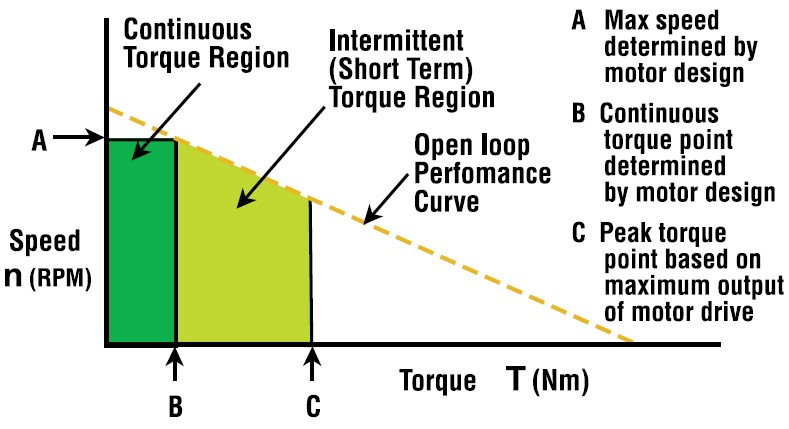Figure 7a. Deriving "System" Performance: Steady State Temperature Rise & System Limitations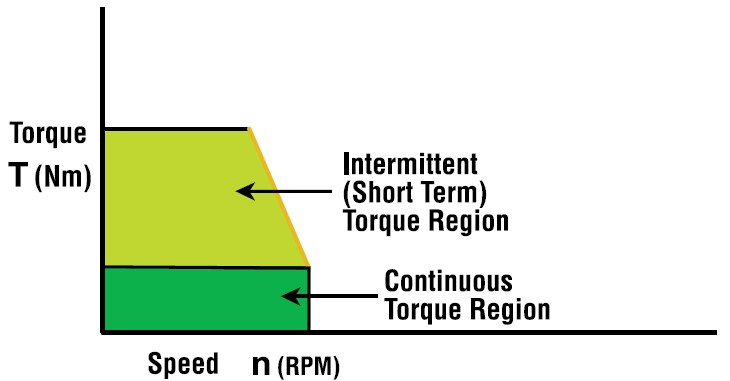Figure 7b. Torque and Speed Axis of Figure 7a Reversed

Another factor to keep in mind when evaluating various performance curves is that actual test conditions may not always be obvious. Different suppliers will publish different information. In the case where the motors are tested rapidly on a dynamometer (to minimize temperature rise), this method tends to be very consistent and provides a good baseline. The disadvantage is that the results don’t represent worst case conditions.

If the motors are tested at the maximum rated temperature, the user will better understand the motor capability when used in applications where motor temperature will stabilize at a value significantly higher than room temperature. The disadvantage is that there are many other variables that can skew the test results such as method of temperature measurement, motor mounting (causing a heat sinking effect), air flow around the motor, etc. Some motor manufacturers will test their motors under the worst case condition; a motor stabilized to full rated temperature with no heat sinking or forced air flow. There are no strict guidelines dictating how a manufacturer should present the performance data. The important thing to remember when evaluating the information is to ask the right questions.Overall Motor Construction and Heat Transfer
Mechanical structure affects the heat transfer characteristics in the motor. In a DC motor with a mechanical commutator and brushes, the copper windings are wound in slots around the “rotating” part of the motor (called the armature). The heat generated by the copper windings on the armature will conduct through the armature laminations and to the motor shaft and bearing system. Through convection, the heat will also migrate across the air gap to the permanent magnets and housing where it will ultimately be dissipated into the surrounding environment. Although some of the heat transfer takes place using direct conduction through the lamination stack to the bearing system and housing, much of the heat generated will flow via the air gap and through the magnets.

In the case of a brushless DC motor, the copper windings are wound in slots (in a slotted brushless motor) or formed against magnetic back iron (in a slotless brushless motor) and constructed as part of the inside surface of the motor housing. This construction allows a direct thermally conductive path from the heat generating copper to the motor housing where it will be dissipated into the environment. The permanent magnets in a brushless motor are on the rotating part of the machine.

In either case, it will be very difficult if not impossible to predict the temperature rise of individual components without empirical testing, but one thing is certain; the temperature rise of individual components (hot spots within the motor) will be different between a brush and brushless motor, all other factors being equal.There’s No Substitute for Application Testing
The purpose of the preceding discussion and the calculation examples is to gain a better understanding of how motor performance changes with temperature. There is no substitute for testing and validating the motor under application conditions. A proper theoretical treatment would not be feasible due to the number of variables and assumptions involved, however, the example calculations can serve as a first approximation to get an idea of the magnitude of possible change.

The best way to understand motor performance under actual operating conditions is to simulate the application as closely as possible while collecting temperature data over time with a data acquisition system. Various parts of the motor should be monitored using thermocouples attached to bearings, end bells, windings (in the case of a brushless motor), magnets (in the case of a brush motor) and the motor housing. Always discuss your design criteria with an applications engineer.